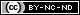[Table of Contents] [Index] [ Version 8 | Version 7 | Version 6 | Version 5 | Version 4 ]

## 3.1 The programming language of OpenFOAM

In order to understand the way in which the OpenFOAM library works, some background knowledge of C++, the base language of OpenFOAM, is required; the necessary information will be presented in this chapter. Before doing so, it is worthwhile addressing the concept of language in general terms to explain some of the ideas behind object-oriented programming and our choice of C++ as the main programming language of OpenFOAM.

### 3.1.1 Language in general

The success of verbal language and mathematics is based on efficiency, especially in expressing abstract concepts. For example, in fluid flow, we use the term “velocity field”, which has meaning without any reference to the nature of the flow or any specific velocity data. The term encapsulates the idea of movement with direction and magnitude and relates to other physical properties. In mathematics, we can represent velocity field by a single symbol, e.g., and express certain concepts using symbols, e.g. “the field of velocity magnitude” by. The advantage of mathematics over verbal language is its greater efficiency, making it possible to express complex concepts with extreme clarity.

The problems that we wish to solve in continuum mechanics are not presented in terms of intrinsic entities, or types, known to a computer, e.g. bits, bytes, integers. They are usually presented first in verbal language, then as partial differential equations in 3 dimensions of space and time. The equations contain the following concepts: scalars, vectors, tensors, and fields thereof; tensor algebra; tensor calculus; dimensional units. The solution to these equations involves discretisation procedures, matrices, solvers, and solution algorithms.

### 3.1.2 Object-orientation and C++

Progamming languages that are object-oriented, such as C++, provide the mechanism — classes — to declare types and associated operations that are part of the verbal and mathematical languages used in science and engineering. Our velocity field introduced earlier can be represented in programming code by the symbol U and “the field of velocity magnitude” can be mag(U). The velocity is a vector field for which there should exist, in an object-oriented code, a vectorField class. The velocity field U would then be an instance, or object, of the vectorField class; hence the term object-oriented.

The clarity of having objects in programming that represent physical objects and abstract entities should not be underestimated. The class structure concentrates code development to contained regions of the code, i.e. the classes themselves, thereby making the code easier to manage. New classes can be derived or inherit properties from other classes, e.g.  the vectorField can be derived from a vector class and a Field class. C++ provides the mechanism of template classes such that the template class Field<Type> can represent a field of any <Type>, e.g. scalar, vector, tensor. The general features of the template class are passed on to any class created from the template. Templating and inheritance reduce duplication of code and create class hierarchies that impose an overall structure on the code.

### 3.1.3 Equation representation

A central theme of the OpenFOAM design is that the solver applications, written using the OpenFOAM classes, have a syntax that closely resembles the partial differential equations being solved. For example the equationis represented by the code

solve
(
fvm::ddt(rho, U)
+ fvm::div(phi, U)
- fvm::laplacian(mu, U)
==
);
This and other requirements demand that the principal programming language of OpenFOAM has object-oriented features such as inheritance, template classes, virtual functions and operator overloading. These features are not available in many languages that purport to be object-orientated but actually have very limited object-orientated capability, such as FORTRAN-90. C++, however, possesses all these features while having the additional advantage that it is widely used with a standard specification so that reliable compilers are available that produce efficient executables. It is therefore the primary language of OpenFOAM.

### 3.1.4 Solver codes

Solver codes are largely procedural since they are a close representation of solution algorithms and equations, which are themselves procedural in nature. Users do not need a deep knowledge of object-orientation and C++ programming to write a solver but should know the principles behind object-orientation and classes, and to have a basic knowledge of some C++ code syntax. An understanding of the underlying equations, models and solution method and algorithms is far more important.

There is often little need for a user to immerse themselves in the code of any of the OpenFOAM classes. The essence of object-orientation is that the user should not have to go through the code of each class they use; merely the knowledge of the class’ existence and its functionality are sufficient to use the class. A description of each class, its functions etc. is supplied with the OpenFOAM distribution in HTML documentation generated with Doxygen at https://cpp.openfoam.org